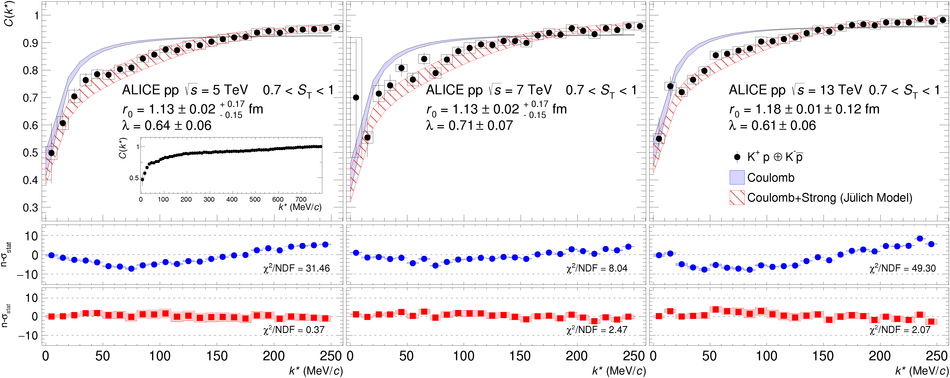# Scattering studies with low-energy kaon-proton femtoscopy in proton-proton collisions at the LHC

The study of the strength and behaviour of the antikaon-nucleon ($\mathrm{\overline{K}N}$) interaction constitutes one of the key focuses of the strangeness sector in low-energy Quantum Chromodynamics (QCD). In this letter a unique high-precision measurement of the strong interaction between kaons and protons, close and above the kinematic threshold, is presented. The femtoscopic measurements of the correlation function at low pair-frame relative momentum of (K$^+$ p $\oplus$ K$^-$ $\overline{\mathrm{p}}$) and (K$^-$ p $\oplus$ K$^+$ $\overline{\mathrm{p}}$) pairs measured in pp collisions at $\sqrt{s}$ = 5, 7 and 13 TeV are reported. A structure observed around a relative momentum of 58 MeV/$c$ in the measured correlation function of (K$^-$ p $\oplus$ K$^+$ $\overline{\mathrm{p}}$) with a significance of 4.4. $\sigma$ constitutes the first experimental evidence for the opening of the $(\mathrm{\overline{K}^0 n} \oplus \mathrm{K^0 \overline{n}})$ isospin breaking channel due to the mass difference between charged and neutral kaons. The measured correlation functions have been compared to J\"{u}lich and Kyoto models in addition to the Coulomb potential. The high-precision data at low relative momenta presented in this work prove femtoscopy to be a powerful complementary tool to scattering experiments and provide new constraints above the $\mathrm{\overline{K}N}$ threshold for low-energy QCD chiral models.

Figures

## Figure 1

 (\kp p \opl\ \km \ap) correlation functions obtained from pp collisions at \s~=~5~TeV (left), 7 TeV (middle) and 13 TeV (right). The inset shows the correlation function evaluated for pp collisions at \s~=~5~TeV in a wider \kstar interval. The measurement is shown by the black markers, the vertical lines and the boxes represent the statistical and systematic uncertainties respectively. Bottom panels represent comparison with models as described in the text.## Figure 2

 (\km p \opl\ \kp \ap) correlation functions obtained (from left to right) from pp collisions at \s~=~5~TeV, 7 TeV, 13 TeV. The fourth panel shows the combined results at the three colliding energies, The number of pairs in each data sample as been used as weight. The inset shows the correlation function evaluated for pp collisions at \s~=~5~TeV in a wider \kstar interval. The measurement is presented by the black markers, the vertical lines and the boxes represent the statistical and systematic uncertainties respectively. Bottom panels represent comparison with models as described in the text.